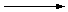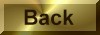Practice Problem 8

Explain why an 0.100 m solution of HCl dissolved in benzene has a freezing point depression of 0.512oC, while an 0.100 m solution of HCl in water has a freezing point depression of 0.352oC.

We can predict the change in the freezing point that should occur in these solutions from the freezing point depression constant for the solvent and the molality of the solution. For benzene, the results of this calculation agree with the experimental value.TFP = -kfm = -(5.12oC/m)(0.100 m) = -0.512oC

For water, however, this calculation gives a predicted value for the freezing point depression that is half of the observed value.TFP = -kfm = -(1.853oC/m)(0.100 m) = -0.185oC

In order to explain these results, it is important to remember that colligative properties depend on the relative number of solute particles in a solution, not their identity. If the acid dissociates to an appreciable extent, the solution will contain more solute particles than we might expect from its molality.

If HCl dissociates completely in water, the total concentration of solute particles (H3O+ and Cl- ions) in the solution will be twice as large as the molality of the solution. The freezing point depression for this solution therefore will be twice as large as the change that would be observed if HCl did not dissociate.

HCl(g) + H2O(l)H3O+(aq) + Cl-(aq)

If we assume that 0.100 m HCl dissociates to form H3O+ and Cl- ions in water, the freezing point depression for this solution should be -0.371oC, which is slightly larger than what is observed experimentally.TFP = -kfm = -(1.853oC/m)(2 x 0.100 m) = -0.371oC

This exercise suggests that HCl does not dissociate into ions when it dissolves in benzene, but dilute solutions of HCl dissociate more or less quantitatively in water.